# PSAT Math : How to multiply decimals

## Example Questions

### Example Question #1 : How To Multiply Decimals

45.728 x 3.2 = ?

14632.96

146.3296

14.63296

146329.6

1463.296

146.3296

Explanation:

Multiply the numbers out in long format, then move the decimal point over the total number of decimal points in the two numbers (3 in the first, 1 in the second, so 4 total):

45.728 * 3.2 = 91456

91456 + 1371840 = 1,463,296 → 146.3296

Or just count the decimal points in the answer and make sure it's 4.

### Example Question #2 : How To Multiply Decimals

At the farmer's market, oranges are $0.30 each, apples are$0.25 each, bananas are $0.40 each, and tomatoes are$0.60 each. If Scott buys 3 oranges, 7 apples, 4 bananas, and 8 tomatoes, how much does he spend?

$9.05$7.75

$6.80$10.40

$11.40 Correct answer:$9.05

Explanation:

To find out how much Scott spends, we need to multiply the quantity of each fruit by its price and add them all up.

3 * $0.30 + 7 *$0.25 + 4 * $0.40 + 8 *$0.60 = $9.05 ### Example Question #3 : How To Multiply Decimals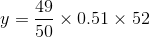Ifis 25% of, what is the closest integer to the value of? Possible Answers: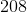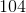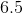Correct answer:Explanation: We can approximate the value of y pretty quickly: y ≈ 1(0.50)(52) = 26 Since y = 0.25x = (1/4)x we know that x = 4y ≈ 4(26) = 104 ### Example Question #4 : How To Multiply Decimals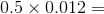Possible Answers: 6 0.0006 0.06 0.6 0.006 Correct answer: 0.006 Explanation: It's much easier to work with whole numbers, so we'll ignore the decimals for the first step: 5 x 12 = 60 Now look at the decimals. To add them back in, think of 60 as 60.0. Now move the decimal point four places to the left: 0.5 x 0.012 = .0060 = .006 ### Example Question #1 : Decimal Operations At the store, green fabric costs$4.53 a yard. How much does 3.8 yards of green fabric cost? (Round to two decimal places.)

$17.21$17.12

$17.13$17.22

$17.31 Correct answer:$17.21

Explanation:

This is a simple multiplication problem involving decimals. Simply multiply 4.53 by 3.8: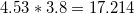Now, round to the nearest two decimal places, giving an answer of 17.21.

### Example Question #2 : Decimal Operations

Find the product. Round to the nearest hundredths place.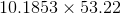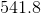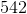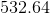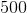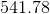The hundredths place is the position two places to the right of the decimal. Our product is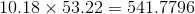. Rounding to the hundredths place results in.